# Balancing redox reaction:

Redox reaction can be balanced by either of the following two methods:

• Oxidation number method
• Ion-electron method

## Oxidation number method to the balanced redox reaction

The oxidation number of an oxidizing agent decreases and that of the reducing agent increases in a redox process. This change in oxidation number should be balanced to balance a redox reaction by the oxidation number method.

Balancing of redox reaction by oxidation number method involves the following steps:

### Step I: Identifying the oxidizing and reducing agents.

Oxidizing and reducing agents are identified by observing the change in oxidation number of main elements present in oxidizing and reducing agents on reactant and product sides. Example:

Here, Zn is oxidized and HNO3 is reduced to Zn(NO3)2 and N2O respectively.

So, Zn is a reducing agent and HNO3 is an oxidizing agent.

### Step II: Calculate the change in oxidation number.

The total change in oxidation number in oxidation and also in reduction is calculated after equalizing the number of atoms undergoing O.N. change on both sides. Example:

Oxidation: total change in O.N. = 2-0 = 2

Reduction: Total change in O.N. = 1-5 = 4×2 =8

### Step III: Balancing of oxidizing and reducing agents.

The number of atoms undergoing oxidation number change is balanced by criss-cross multiplication with change in oxidation number on reactant as well as product side. Example.

### Step IV: Balancing of other elements.

All the other atoms or radicals are balanced by the hit and trial method.

• Balancing the equation with respect to all other atoms except hydrogen and oxygen.

It is the balanced chemical equation.

Note:
• For reactions taking place in acidic solutions, add H+ ions to the side deficient in hydrogen atoms.
• For reactions taking place in basic solutions, add H2O molecules to the side deficient in hydrogen atoms and simultaneously add an equal number to OH– ions on the other side of the equation.

Example – 1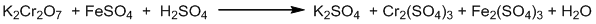Step I: Identifying the oxidizing and reducing agents.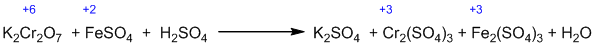Step II: Calculate the change in oxidation number.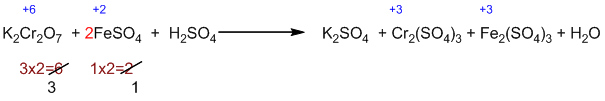Step III: Balancing of oxidizing and reducing agents.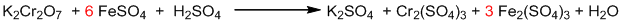Step IV: Balancing of other elements.

• Balancing the equation with respect to all other atoms except hydrogen and oxygen.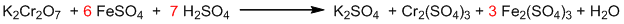• Finally, balancing hydrogen and oxygen.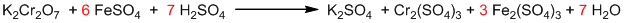It is the balanced chemical equation.

Example – 2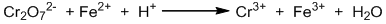Step I: Identifying the oxidizing and reducing agents.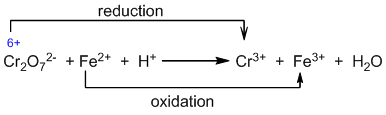Step II: Calculate the change in oxidation number.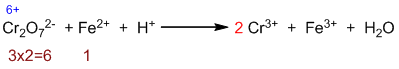Step III: Balancing of oxidizing and reducing agents.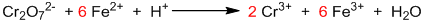Step IV: Balancing of other elements.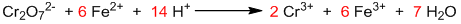It is the balanced chemical equation.

Example-3 :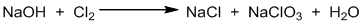Step I: Identifying the oxidizing and reducing agents.

Here, Cl2 undergoes self oxidation and reduction (i.e. disproportionation reaction). So, Cl2 is written twice to calculate the change in oxidation number.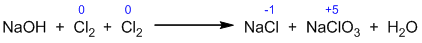Step II: Calculate the change in oxidation number.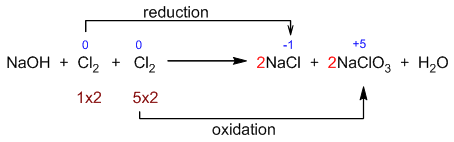Step III: Balancing of oxidizing and reducing agents.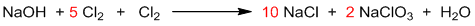Step IV: Balancing of other elements.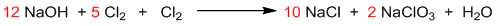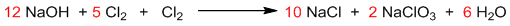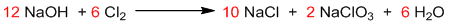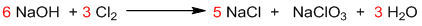It is the balanced chemical equation.

Example-4 :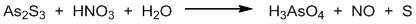Step I: Identifying the oxidizing and reducing agents.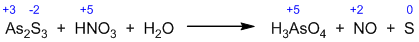Step II: Calculate the change in oxidation number.

Here, more than two elements have changed in the oxidation number.

Change in O.N. of As = +2 X 2 = +4

Change in O.N. of S = +2 X 3 = +6

Change in O.N. of N = – 3

Thus, total increase in O.N. = 4+6=10

Total decrease in O.N. = 3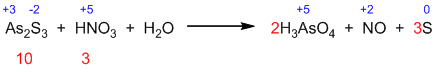Step III: Balancing of oxidizing and reducing agents.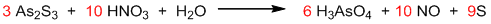Step IV: Balancing of other elements.

It is the balanced chemical equation.

## Ion-Electron method [ Half reaction method] to balance reaction

This method is based on the fact that the number of electrons lost during the oxidation half-reaction of a redox reaction is equal to the number of electrons gained during the reduction half-reaction.

Following steps are involved in balancing redox reaction by the ion-electron method:

• Write the given equation and identify the oxidized and reduced species by analyzing changes in oxidation number.
• Write the oxidation and reduction half-reactions (ionic if required)
• Balance two half-reactions separately.
1. Balance main element (i.e. atom whose O.N. is changed) by hit and trial.
2. Balance oxygen by adding H2O.
3. Balance hydrogen by adding H+.
4. Balance charge by adding electrons.
5. In a basic medium, OH ions are added on either side to cancel out H+ ions.
• Add two half-reactions by making electrons gained equal to electrons lost in two half-reactions.
• Finally, convert the balanced ionic equation to a balanced molecular equation (if required).

Some Examples:

Example-1 :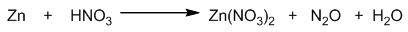Counting of oxidation numbers: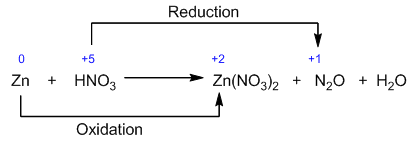Identification and balancing of two half-reactions:

Oxidation half-reaction: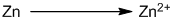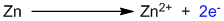This is a balanced oxidation half-reaction.

Reduction half-reaction: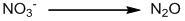Balancing the main element by hit and trial: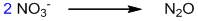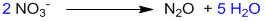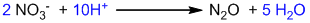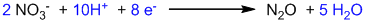This is a balanced reduction half-reaction.

Balanced two half-reactions are added after multiplying oxidation half by 4 to cancel electrons.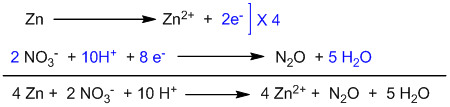This is the balanced ionic equation. To convert it into molecular form, 8 NO3 ions are added on both sides of the equation.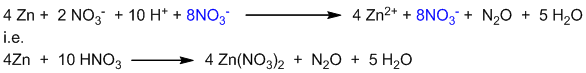This is the balanced molecular equation.

Example-2: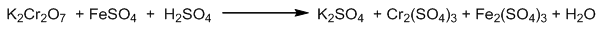Counting of oxidation numbers: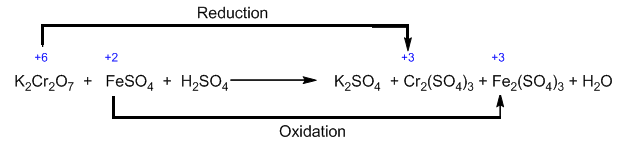Identification and balancing of two half-reactions:

Oxidation half-reaction: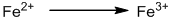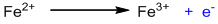This is a balanced oxidation half-reaction.

Reduction half-reaction: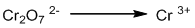Balancing the main element by hit and trial: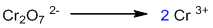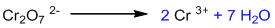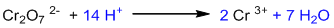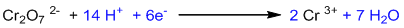This is a balanced reduction half-reaction.

Balanced two half-reactions are added after multiplying oxidation half by 6 to cancel electrons.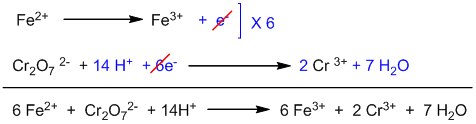This is the balanced ionic equation. To convert it into molecular form, suitable numbers of K+ and SO42- have to be added on both sides of the equation.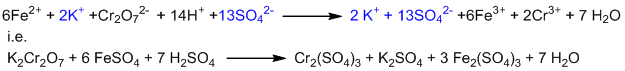This is the balanced molecular equation.

Example -3: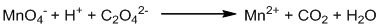Counting of oxidation numbers: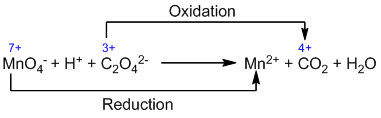Identification and balancing of two half-reactions:

Oxidation half-reaction: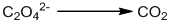Balancing the main element by hit and trial: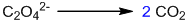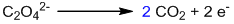This is a balanced oxidation half-reaction.

Reduction half-reaction: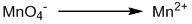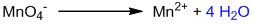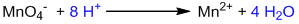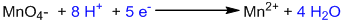This is a balanced reduction half-reaction.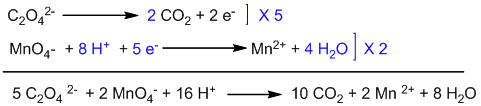This is a balanced equation.

Example-4: [ Basic medium ]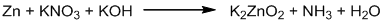Counting of oxidation number: Here, chlorine is oxidized as well as reduced.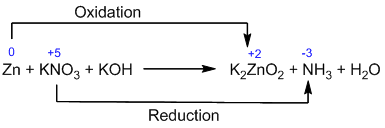Identification and balancing of two half-reactions:

Oxidation half-reaction: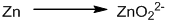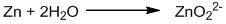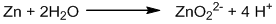The reaction is carried out in a basic medium, so, all H+ ions must be neutralized by adding the same number of OH ions on both sides.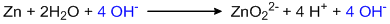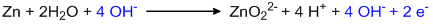This is a balanced oxidation half-reaction.

Reduction half-reaction: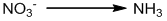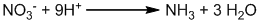The reaction is carried out in a basic medium, so, all H+ ions must be neutralized by adding the same number of OH ions on both sides.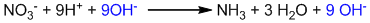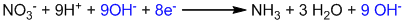This is a balanced reduction half-reaction.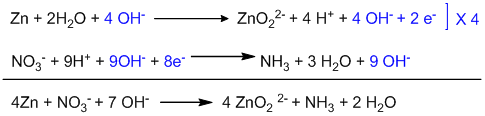This is the balanced ionic equation. In order to convert it into molecular form, 8K+ ions are added on both sides of the equation.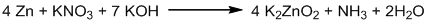This is the balanced molecular equation.

Example-5: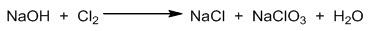Counting of oxidation number: Here, chlorine is oxidized as well as reduced.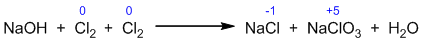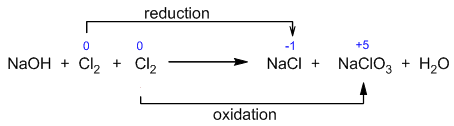Identification and balancing of two half-reactions:

Oxidation half-reaction: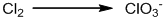Balancing the main element by hit and trial: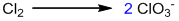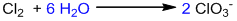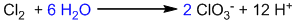The reaction is carried out in a basic medium, so, all H+ ions must be neutralized by adding the same number of OH ions on both sides.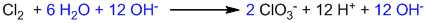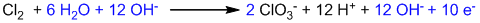This is a balanced oxidation half-reaction.

Reduction half-reaction: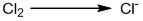Balancing the main element by hit and trial: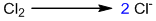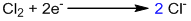This is a balanced reduction half-reaction.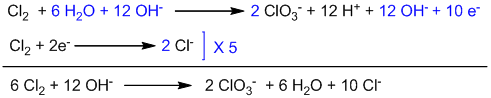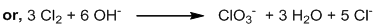This is the balanced ionic equation. In order to convert it into molecular form, 6 Na+ ions are added on both sides of the equation.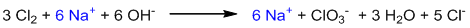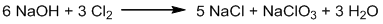This is the balanced molecular equation.

#### Self Practice:

##### Balance the following equations by the oxidation number method or ion-electron method.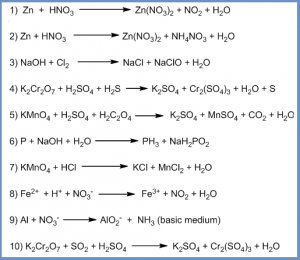Reactions